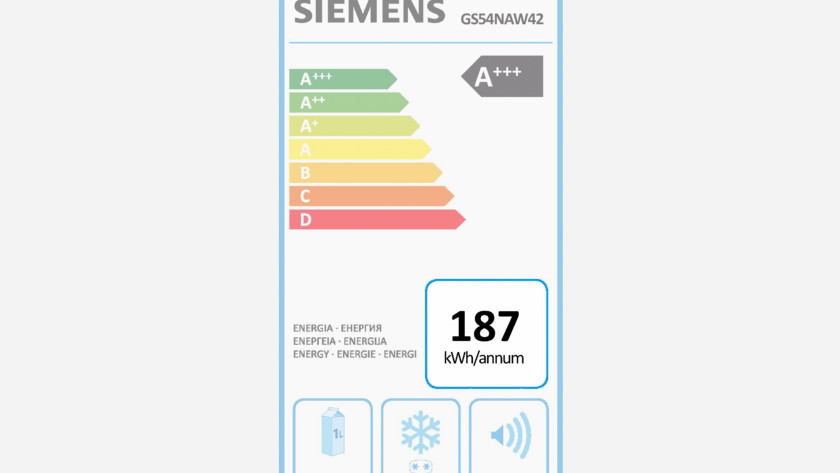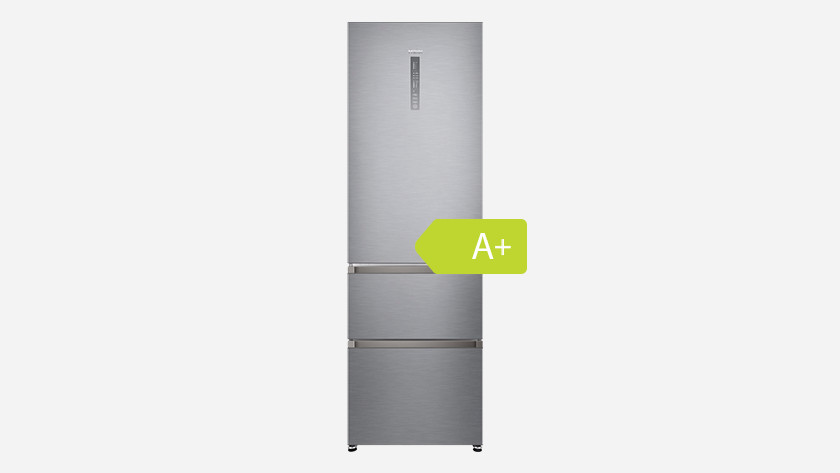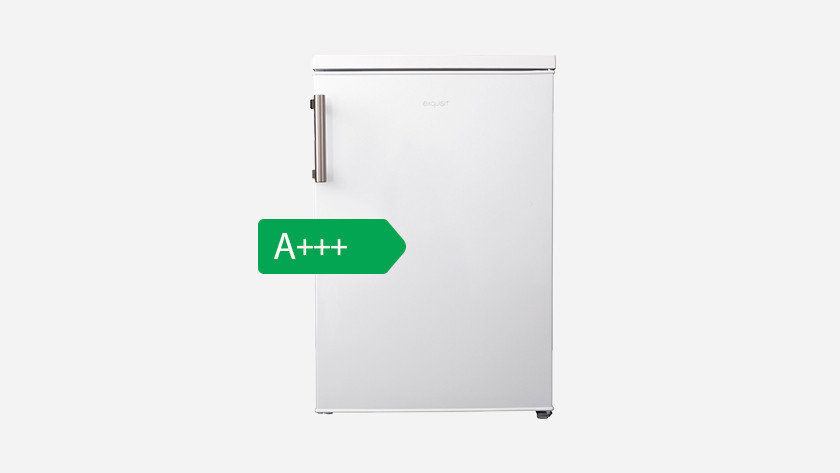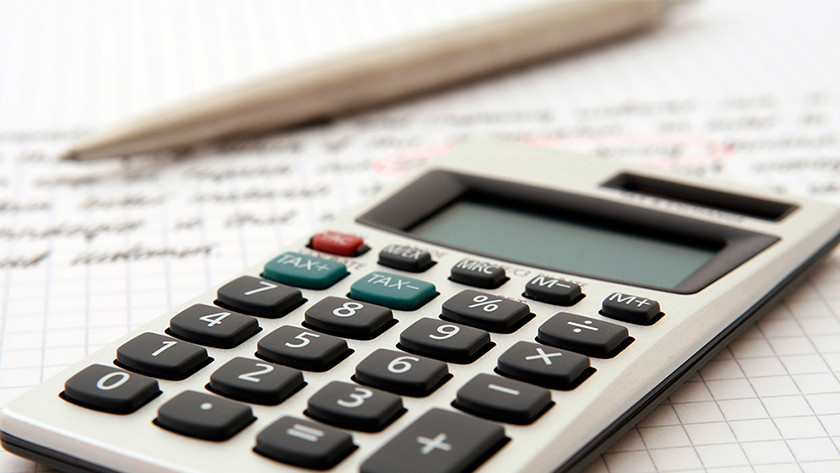Written by Lieze

# How much energy does a fridge use?

Fridges are energy savers. They're on day and night and remain active to keep the air cool in the fridge. In order to avoid unexpectedly high bills, it's nice if you know the energy consumption and the energy costs. In this article, you can read what the consumption of a fridge is and how you calculate the energy costs.

## Average consumption of refrigerator per year

Fridge freezer combination Tabletop fridge 1-door fridge
A+++ 164 kWh 82 kWh 74 kWh
A++ 228 kWh 121 kWh 116 kWh
A+ 273 kWh 138 kWh 139 kWh

## Average energy costs per year

Fridge freezer combination Tabletop fridge 1-door fridge
A+++ € 37.76 € 18.91 € 17.35
A++ € 52.51 € 27.73 € 26.74
A+ € 62.87 € 31.90 € 32.04

## The exact consumption is shown on the energy labelThe energy label indicates the average energy consumption of a fridge in kilowatt hours (kWh). This wattage tells you the average power consumption of the fridge per year. The power consumption is also related to the energy label of the fridge, which is somewhere between A+++ and A+. While an A+ fridge may seem economical, it actually uses the most energy. Fridges with an A+++ energy label use the least amount of energy.

## Fridge-freezer: saving A +++ vs. A +With an energy-efficient A +++ fridge-freezer combination you save € 177, - on energy costs and the CO2 emissions of a car ride of 10,000 kilometers. An A +++ fridge-freezer combination is therefore better for your wallet and the environment.

## Table model: saving A +++ vs. A +Choosing an energy-efficient table model has two advantages. Due to the lower energy costs you save € 100 after 15 years and the CO2 emissions of a flight to Marrakesh.

## Calculate energy costsTo calculate the energy costs in euros per year, you do the following. Read the average energy consumption in kilowatt hours (kWh) on the energy label. Multiply this number by € 0.23. This is the average energy price in the Netherlands. Then you get the following formula:

** € 0.23 x average energy consumption in kWh per year (see energy label) = energy costs per year **Article by:
Lieze Fridge Expert.

© 1999 - 2020 - Coolblue B.V.
Customer rating: 9.2 / 10 -